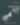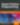# AtoZ CSS Quick Tip: Using Y-Axis Transforms and Matrices

HTML & CSS

Share:

#### Free JavaScript Book!Write powerful, clean and maintainable JavaScript.

RRP \$11.95

You can view the full transcript and screencast on `rotateY` here.

Welcome to our AtoZ CSS series! In this series, I’ll be exploring different CSS values (and properties) each beginning with a different letter of the alphabet. We know that sometimes screencasts are just not enough, so in this article, we’ve added a quick tip on Y-Axis transforming and matrices.## Y is for Y-Axis transforming and matrices

We can perform all sorts of interesting transformations on elements along various different axis. In this tip, we’ll provide a rundown of all the common options and then look at a lesser known value of `transform` which allows us to set multiple `transform` values via the `matrix()` value.

### `transform` along the y-axis

Since the original screencast focused around `rotateY()`, I thought it would be useful to look at all other available transformations that happen along the vertical y-axis.

We can apply the following transformations:

• `transform: translateY(100px)`
• `transform: rotateY(45deg)`
• `transform: scaleY(1.5)`
• `transform: skewY(30deg)`

These values are all limited to the y-axis and can be used to communicate intent that the only transformation along this single axis is being performed.

`rotateY()` is the only one of these values that performs a 3D transformation, where the element is rotated around the y-axis. If performing any kind of 3D `transform`, be sure to set `transform-style:preserve-3d` on the parent element.

### Multiple `transform`

If you want to combine multiple `transform` values together, this can be done by space separating the different transformation functions. The following snippet combines a translation with a `skew` for example:

``````.element {
transform: translateY(50px) skew(30deg);
}``````

This is all well and good but if you are setting multiple `transform` like this as part of an animation or transition, it can be quite fiddly as every part of the `transform` needs to be addressed in each state or keyframe.

There is a slightly more complex but much more powerful method for setting `transform` via the `matrix()` value.

### Set complex `transform` with a `matrix()`

The `matrix()` is a single value that allows you to set `scale`, `skew` and `translate` in one go by passing 6 numeric parameters.

``````.element {
transform: matrix(a, b, c, d, e, f);
}``````

At the heart of this technique is some pretty complex maths which has always hurt my brain to try and figure out. So instead of me trying to explain mathematical matrices, dot products, and vectors, let’s have a look at what each of the parameters in the `matrix()` refer to.

If you do want a full on deep dive into the maths, have a read of this article by User Agent Man.

In the above snippet, I’ve labeled each argument to the matrix function with a different letter from `a` to `f`.

`a` and `d` are used to set the horizontal and vertical scale.

`b` and `c` are used to set the amount of horizontal and vertical skew.

Finally, `e` and `f` are for setting the horizontal and vertical translation.

One thing that makes this whole `matrix()` thing a bit hard to grasp is that each argument is provided as a number value rather than a length. Lengths are values like `100px` or `30deg` but these aren’t valid values for a matrix and we need to use numbers instead.

To demonstrate this, the snippet below produces two identical visual results; one using multiple `transform` and on with a single matrix:

``````.one {
transform: scale(1.5, 1.5) skew(10deg, 10deg) translate(100px, -50px);
}
.two {
transform: matrix(1.5, 0.26449, 0.26449, 1.5, 136.775, -48.551);
}``````

Here’s a Codepen of the above snippet so you can see the identical result:

See the Pen qqEjMz by SitePoint (@SitePoint) on CodePen.

As much as the `matrix()` value is useful for setting many values in one go, it’s not particularly human readable. It’s great if you’re doing a lot of JavaScript maths to work out all the various component parts of the matrix but it’s very confusing to write by hand.

If you want to dig into matrices further then you can “Inspect Element” on any element that’s been transformed and look at the “computed styles” tab. The matrix for the `transform` being applied will be shown in full.### Guy Routledge

Front-end dev and teacher at The General Assembly London. A to Z CSS Screencaster, Founder of sapling.digital and Co-founder of The Food Rush.

#### New books out now!Learn how Git works, and how to use it to streamline your workflow!Google, Netflix and ILM are Python users. Maybe you should too?